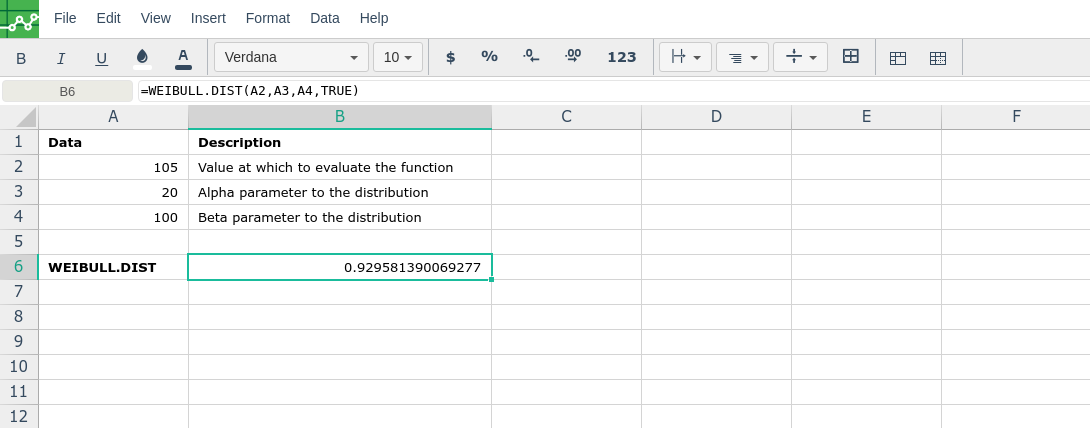# WEIBULL.DIST

### Definition

Returns the Weibull distribution. Use this distribution in reliability analysis, such as calculating a device's mean time to failure.

### Syntax

WEIBULL.DIST(x,alpha,beta,cumulative)

The WEIBULL.DIST function syntax has the following arguments:

• X     Required. The value at which to evaluate the function.

• Alpha     Required. A parameter to the distribution.

• Beta     Required. A parameter to the distribution.

• Cumulative     Required. Determines the form of the function.

### Remarks

• If x, alpha, or beta is nonnumeric, WEIBULL.DIST returns the #VALUE! error value.

• If x < 0, WEIBULL.DIST returns the #NUM! error value.

• If alpha ≤ 0 or if beta ≤ 0, WEIBULL.DIST returns the #NUM! error value.

• The equation for the Weibull cumulative distribution function is:

• The equation for the Weibull probability density function is:

• When alpha = 1, WEIBULL.DIST returns the exponential distribution with:

###Error.

User does not have sufficient privileges to access this Content